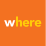# Batch convert .las files to .slpk

519
10
04-20-2021 11:27 PM
Labels (1)New Contributor

I cannot seem to figure out a way to batch convert several LAS files to the SLPK file type. I have tried both creating a model using model builder but the 'Create Point Cloud Scene Layer Package' tool and the 'Iterate Files' iterator, but the tool will not accept the input of the files from the iterator (reference 'Model' images below). I have also tried to create a python script and run it using the notebooks option in ArcGIS Pro and am able to have multiple .las files convert into a single .slpk file but I cannot get the script to output a single .slpk for each .las (reference 'Script_error' image below). Any help would be great, I have exhausted my online resources.

Script of multiple .las to a single .slpk:

import arcpy
import os

from arcpy import env
arcpy.env.overwriteOutput = True

wksp = env.workspace = "C:\LiDARTest"

inpath = "C:\LiDARTest\InFile"
outpath = "C:\LiDARTest\OutFile"

Output_Coordinate_System="PROJCS['NAD_1983_2011_StatePlane_Illinois_East_FIPS_1201_Ft_US',GEOGCS['GCS_NAD_1983_2011',DATUM['D_NAD_1983_2011',SPHEROID['GRS_1980',6378137.0,298.257222101]],PRIMEM['Greenwich',0.0],UNIT['Degree',0.0174532925199433]],PROJECTION['Transverse_Mercator'],PARAMETER['False_Easting',984250.0],PARAMETER['False_Northing',0.0],PARAMETER['Central_Meridian',-88.33333333333333],PARAMETER['Scale_Factor',0.999975],PARAMETER['Latitude_Of_Origin',36.66666666666666],UNIT['Foot_US',0.3048006096012192]];-17463800 -46132600 3048.00609601219;-100000 3048.00609601219;-100000 10000;3.28083333333333E-03;3.28083333333333E-03;0.001;IsHighPrecision"

arcpy.management.CreatePointCloudSceneLayerPackage(in_dataset=inpath, out_slpk=outpath, out_coor_system=Output_Coordinate_System, transform_method=[], attributes=["INTENSITY", "RGB", "CLASS_CODE", "FLAGS", "RETURNS"], xy_max_error_m=0.01, z_max_error_m=0.01, in_coor_system="", scene_layer_version="2.x")

Script of multiple .las to multiple .slpk:

import arcpy
import os

from arcpy import env
arcpy.env.overwriteOutput = True

wksp = env.workspace = "C:\LiDARTest"

rootdir = "C:\LiDARTest\InFile"

name = 0
Output_Coordinate_System="PROJCS['NAD_1983_2011_StatePlane_Illinois_East_FIPS_1201_Ft_US',GEOGCS['GCS_NAD_1983_2011',DATUM['D_NAD_1983_2011',SPHEROID['GRS_1980',6378137.0,298.257222101]],PRIMEM['Greenwich',0.0],UNIT['Degree',0.0174532925199433]],PROJECTION['Transverse_Mercator'],PARAMETER['False_Easting',984250.0],PARAMETER['False_Northing',0.0],PARAMETER['Central_Meridian',-88.33333333333333],PARAMETER['Scale_Factor',0.999975],PARAMETER['Latitude_Of_Origin',36.66666666666666],UNIT['Foot_US',0.3048006096012192]];-17463800 -46132600 3048.00609601219;-100000 3048.00609601219;-100000 10000;3.28083333333333E-03;3.28083333333333E-03;0.001;IsHighPrecision"

for subdirs, dirs, files in os.walk(rootdir):
for file in files:
output_layer = "C:\\LiDARTest\\OutFile\\" + str(name)
arcpy.management.CreatePointCloudSceneLayerPackage(in_dataset=file, out_slpk=output_layer, out_coor_system=Output_Coordinate_System, transform_method=[], attributes=["INTENSITY", "RGB", "CLASS_CODE", "FLAGS", "RETURNS"], xy_max_error_m=0.01, z_max_error_m=0.01, in_coor_system="", scene_layer_version="2.x")
name = name+1

*ignore my naming convention have not taken the time to work on that part of the script

10 RepliesbyEsri Contributor

Hi

Yes, that's probably best at this point 🙂 I'm not sure what time zone you are in but i'm in the pacific time zone. My morning tomorrow is full of meetings but after 12pm i'm free and I can send you a zoom link. Let me know what works for you.

thanks,

Andrew